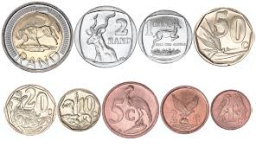One package of chocolate costs 9 CZK more than one package of gingerbread. Five packages of chocolate were CZK 15 more expensive than 10 packages of gingerbread. How much was a package of chocolates, and how much was a package of gingerbread?

x =  15
y =  6

### Step-by-step explanation:

x=9+y
5x = 15 + 10y

x=9+y
5·x = 15 + 10·y

x-y = 9
5x-10y = 15

Pivot: Row 1 ↔ Row 2
5x-10y = 15
x-y = 9

Row 2 - 1/5 · Row 1 → Row 2
5x-10y = 15
y = 6

y = 6/1 = 6
x = 15+10y/5 = 15+10 · 6/5 = 15

x = 15
y = 6

Our linear equations calculator calculates it.Did you find an error or inaccuracy? Feel free to write us. Thank you!

Tips for related online calculators
Do you have a system of equations and looking for calculator system of linear equations?